American Journal of Civil Engineering
Volume 4, Issue 6, November 2016, Pages: 298-305

The Earthquake Ground Motion and Response Spectra Design for Sleman, Yogyakarta, Indonesia with Probabilistic Seismic Hazard Analysis and Spectral Matching in Time Domain

Lalu Makrup, Atika Ulfa Jamal

Department of Civil Engineering, Islamic University of Indonesia, Yogyakarta, Indonesia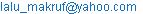(L. Makrup)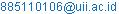(L. Makrup)(A.U. Jamal)

Lalu Makrup, Atika Ulfa Jamal. The Earthquake Ground Motion and Response Spectra Design for Sleman, Yogyakarta, Indonesia with Probabilistic Seismic Hazard Analysis and Spectral Matching in Time Domain. American Journal of Civil Engineering. Vol. 4, No. 6, 2016, pp. 298-305. doi: 10.11648/j.ajce.20160406.15

Received: September 1, 2016; Accepted: September 10, 2016; Published: September 29, 2016

Abstract: Earthquake acceleration time history and response spectra design are needed as basis to determine the earthquake loading that can be used to design and assess the tall building and other structures. The acceleration time history and response spectra design for certain site can be acquired using the seismic hazard analysis, amplification of ground motion quantity, and spectral matching in time domain. The result is the artificial acceleration time history for a site in Sleman District, Yogyakarta, Indonesia. That time history can be utilized as a basis to design and assess the earthquake resistant building structure in the district area.

Keywords: Seismic Hazard, Response Spectra, Amplification, Time Domain, Acceleration Ground Motion

Contents

1. Introduction

To design and assess a building structural to the seismic loading in the future can be utilized earthquake Acceleration Time History (ATH), Figure 2, and or response spectra (RSA) design, Figure 3 and 4. The site of this study is in vicinity of a point with coordinate (110.3531 longitude; -7.7453 latitude) in Sleman District, Yogyakarta Province, Indonesia (Figure 1).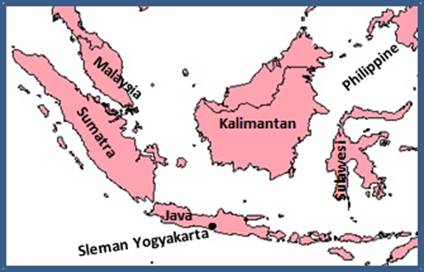Figure 1. Western and Central Indonesia.

Figures 2 and 3 were drawn based on Northridge earthquake, USA 1994 at station MoorPark, with direction 1800 (accessed from http://ngawest2. berkeley.edu/, 27 July 2015).

The ATH such as above (Figure 2), in probabilistic and deterministic procedure can be obtained by four steps of calculation i.e. i) Probabilistic Seismic Hazard Analysis (PSHA), ii) hazard deaggregation, iii) amplification process, and iv) spectra matching. Step i), ii), iii), and spectral matching analysis in time domain was utilized by Irsyam (2003) to carry out seismic hazard assessment for Liquid Natural Gas storage tank terminal of the National Electrical Company (PLN) at Teluk Banten, Western Java, Indonesia. Such steps above as Irsyam (2003) was used also by Aldiamar (2007) to obtain the acceleration time history for Surabaya, Eastern Java i.e. for seismic loading study that used in Suramadu Bridge.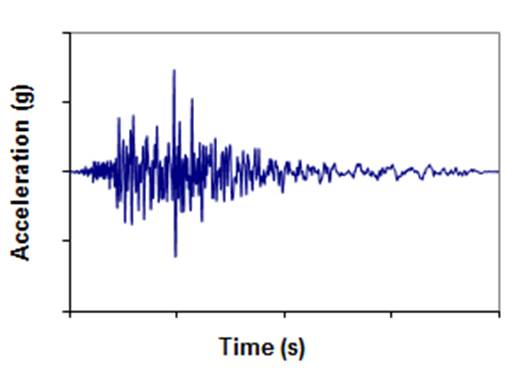Figure 2. Example of actual acceleration time history of an earthquake.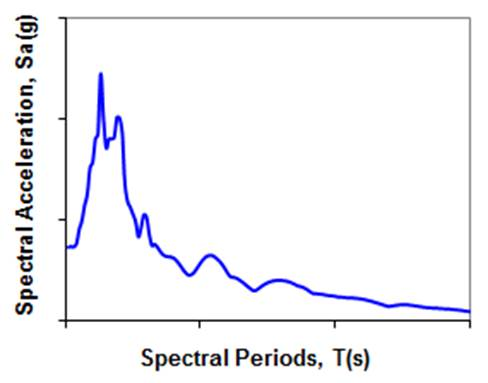Figure 3. Example of actual response spectra of an earthquake with 5% dumping ratio.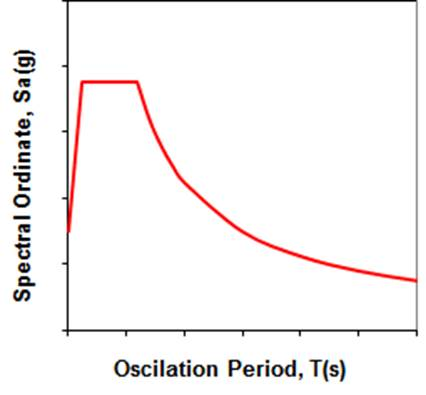Figure 4. Example of response spectra design.

The two authors have carried out calculation to find response spectra design use seismic code of UBC 1997. That code gave the complete procedure to make response spectra design with easy manner.

In this study, four steps above were utilized to obtain the acceleration time history for the site of Sleman, Yogyakarta. The ATH and response spectra design is needed by Sleman district government to design the new tall building and assess the building that already exist there, according to seismic loading.

2. Probabilistic Seismic Hazard Analysis

To acquire the seismic hazard value for Sleman can be utilized Probabilistic Seismic Hazard Analysis (PSHA), Kramer (1996). This above analysis was used by McGuire (1996), which assumed the earthquake magnitude M and the source-to-site distance R as a continuous independent random variable and by Makrup, at al., (2015) to developed seismic hazard program. The PSHA is started with the mean annual rate of exceedance (λ), that was defined as the integral equation (Kramer, 1996) that is

λX(x) = ν rm PX(x |m,r) fM(x) fR(r) dm dr   (1)

where:

λX(x) = mean annual rate of exceedance of a particular value, x by a ground motion parameter X.

PX(x|m,r) = the probability density function of earthquake parameter x due to earthquake magnitude and source-to-site distance.

fM(m) = the probability density function of magnitude.

fR(r) = the probability density function of source-to-site distance.

ν = average rate of threshold magnitude exceedance, defined as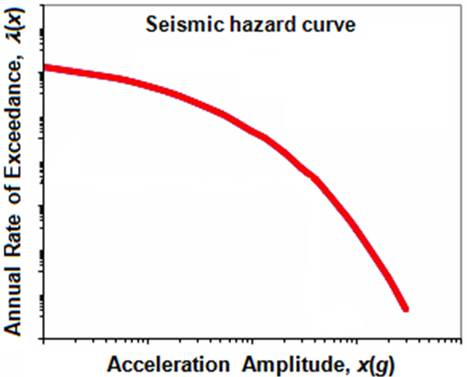Figure 5. Seismic hazard Curve.

ν = 10ab mo                                (2)

Relation between λ(x) and earthquake parameter x call seismic hazard curve (Figure 5) and combination of equation (1) and Poisson process equation (3) gave earthquake parameter (e.g. earthquake ground motion acceleration).

P(X>x) = 1 - e-λ t                                  (3)

In addition, relation between the ground motions and spectral periods are called Uniform Hazard Spectrum (UHS).

2.1. Earthquake Source and Source Model

Probabilistic seismic hazard analysis can be executed with some input that could be done with in the analysis. Earthquake sources, source models, sources parameters, and some another inputs are the essential for the analysis. Sources and source models for the analysis were developed based on Figure 6.

Fault source was represented as individual fault. Parameter of fault source model include: source location (trace coordinate), orientation (strike, deep angle, and maximum depth), faulting style, minimum magnitude, maximum magnitude, magnitude distribution to compute the magnitude probability, and slip rate according to earthquake recurrence interval.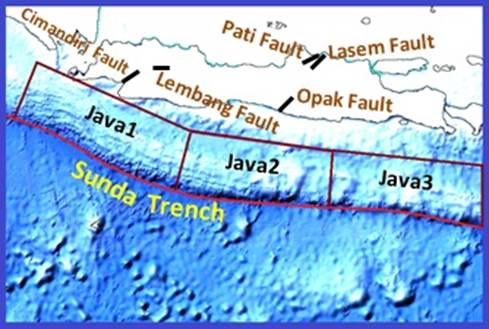Figure 6. Seismic sources in vicinity of Java, Indonesia.

Subduction source was modeled become interface zone with depth up to 50 km and intraplate zone with depth 50 km to 300 km.

The fault parameters that were used for this study are available in Table 1.

Table 1. Faults used in the study.

 Fault Slip Rate Sense Magnitude Name mm/years Mechanism Mmax Cimandiri 4 Strike-Slip 7.9 Yogya 2.4 Strike-Slip 6.5 Lembang 1.5 Strike-Slip 7.3 Pati 0.5 Strike-Slip 6.8 Lasem 0.5 Strike-Slip 7.5 Flores back 28 Reverse- 6.8 Arc slip Subduction b a Mmax Zone parameter parameter Java1 1.10 6.14 8.1 Java 2 1.10 6.14 8.1 Java 3 1.10 6.14 8.1

In the hazard computations, an uncertainty (±MO.2) was applied for the maximum earthquake magnitude. Magnitude minimum for all sources are taken M = 5.

The empirical correlation of Well and Coppersmith (1994) associated with magnitude and rupture length was utilized to calculate the distance and distance probability. Gutenberg-Richter (1944) recurrence model was used to calculate rate of earthquake occurrence.

The truncated exponential magnitude distribution model driven from Gutenberg-Richter (1944) law (weighting 0.66) and characteristic magnitude distribution model of Youngs and Coppersmith (1985) (weighting 0.34) were used to account for the uncertainty in magnitude distribution.

2.2. Ground Motion Model

The ground motion models are referred to as ground motion prediction equations. These models predict the ground motion for a particular fault source, fault type, magnitude, distance, stress drop, attenuation properties of the crust, and local soil condition, (Petersen et al. 2008). Until this day there is no ground motion prediction equation developed for tectonic zone of Indonesia. Therefore for this study is chosen and adapt ground motion prediction equation derived in another region similar to both tectonically and geologically of Indonesia. The selection is carried out based on earthquake mechanism which comprises shallow crustal and subduction zone earthquakes.

To account for uncertainty in ground motion prediction are applied Boore-Atkinson (2007) (weighting 0.5) and Sadigh et al. (1997) (weighting 0.5) for the shallow crustal earthquake sources. The attenuation model of Youngs et al. (1997) and Atkinson-Boore (2003) were adopted to represent the subduction source with scheme weighting 0.5, 0.5 respectively.

3. Hazard Deaggregation Analysis

The hazard deaggregation analysis is employed here to know which earthquakes are contributing most to the hazard in Sleman. The hazard deaggregation analysis was utilized by Makrup (2009) to deaggregate the hazards of some cities in Indonesia.

Hazard that is acquired from section 2, is deaggregated to determine the sources that contribute at hazard levels of 10% probability of exceedance in 50 years. The result is a pair of magnitude and distance that contributed most to the site.

4. Amplification Process

Amplification process is a step to acquire the quantity of a ground motion on soil surface (the soil site condition). The Uniform Building Code (UBC) 1997 is employed to amplify a ground motion in base rock to be a ground motion on soil surface. The result of this amplification is a response spectra design as Figure 4 on soil surface. The UBC 1997 provided formulas and tables to obtain amplification factor and develop the response spectra design. The standard form of UBC1997 spectral is in Figure 7.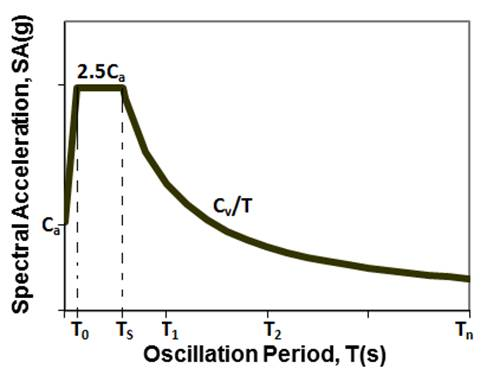Figure 7. Response spectra design standard of UBC 1997.

The procedures to draw the response spectra design Figure 7 are:

i).         Determine the soil site condition (soil type) based on soil layer from investigation result with minimum depth up to 30 m and Table 2. The result of this item is soil type SA or SB etc.

Table 2a. Soil type of the site (UBC 1997).

 Soil Soil N-SPT Type Description SA Hard rock - SB Rock N-SPT > 50 SC Hard soil 15 50 SD Stiff soil 15 50 SE Soft soil N-SPT < 15 SF Special soil -

ii).       Figure out Peak Ground Acceleration (PGA) of base rock in the site based on the PSHA, Section 2.

iii).      Determine the amplification factor FA and FV with z = PGA and soil site condition, Table 3 and 4.

Table 2b. Soil type of the site (UBC 1997).

 Soil Soil Vs(m/s) Type Description SA Hard Rock Vs > 1500 SB Rock 760 < Vs ≤ 1500 SC Hard Soil 350 < Vs ≤ 700 SD Stiff Soil 180 < Vs ≤ 350 SE Soft Soil Vs ≤ 350 SF Special Soil

Table 3. Amplification factor FA of UBC 1997.

 Soil Z z z z z Type 0.075 0.150 0.200 0.300 0.400 SA 0.8 0.8 0.8 0.8 SB 1.0 1.0 1.0 1.0 SC 1.1 1.2 1.2 1.0 SD 1.5 1.5 1.4 1.2 SE 2.4 2.0 1.7 1.2

Table 4. Amplification factor FV of UBC 199.

 Soil Z z z z z Type 0.075 0.150 0.200 0.300 0.400 SA 0.8 0.8 0.8 0.8 SB 1.0 1.0 1.0 1.0 SC 1.6 1.7 1.6 1.5 SD 2.3 2.1 2.0 1.8 SE 3.3 3.3 3.2 2.8

iv).     Finally compute CA, CV TS and T0 use equation below and draw the spectra design utilize these variables.

Ca = z FA                                   (4)

Cv = z FV                                   (5)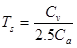(6)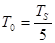(7)

The spectral design is used as a target spectrum in spectral matching process on soil surface.

5. Spectral Matching in Time Domain

Nicolaou (1998) has employed spectral matching in time domain to get the earthquake acceleration time history for a particular site. The procedure that has been used by Nicolaou (1998) was utilized also here to obtain the earthquake acceleration time history for Sleman, Yogyakarta, Indonesia. The procedure is based on minimizing the differences between the target spectrum and ATH response spectrum in a least square sense. The result of that procedure is a scaling factor. For this purpose some parameters that used in spectral matching has to be symbolized (Nicollaou, 1998), that is,

Satarget = target acceleration response spectrum (such as seismic code spectrum, or uniform hazard spectrum, or attenuation law spectrum, or Newmark-Hall spectrum).

Saactuls = acceleration response spectrum of the given (actual) acceleration time history (measured ATH).

α = scaling factor.

T = period of oscillator.

The methodology proposed herein considers as ²error² the squared scale-to-target difference, evaluated by the integral (Nicolaou, 1998),(8)

where TA and TB are the lower and upper period response spectra respectively. To minimize the error, the first derivative of the error function according the scaling factor α has to be zero.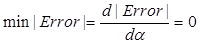(9)

which gives: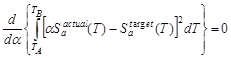(10)

or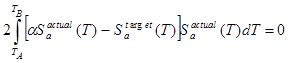(11)

If the equation above is solved respect to the scaling factor, the scaling factor α will be got (equation 12 and 13).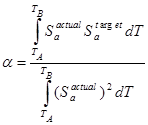(12)

Or, in a discrete form: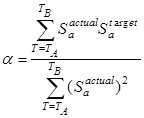(13)

Spectral matching result is the new ATH from the actual ATH multiple by scaling factor α.

6. Result

6.1. Result of Seismic Hazard Calculations

Results of probabilistic seismic analysis for Sleman in rock site (base rock) are Peak Ground Acceleration (PGA) 0.1976g and the uniform hazard spectrum (Figure 8) with level hazard 10% probability of exceedance in 50 years.

PGA = 0.1976g in base rock was used as a basis to execute hazard deaggregation analysis and amplification process by UBC 1997.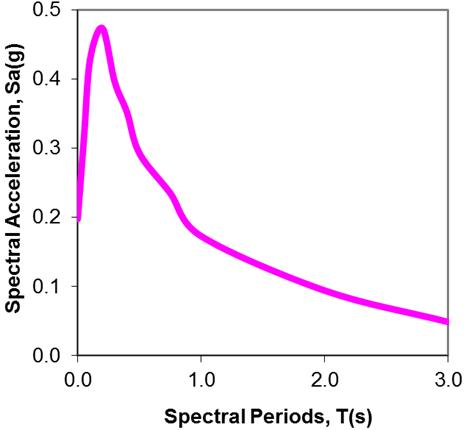Figure 8. Uniform hazard spectrum of Sleman.

6.2. Result of Hazard Deaggregation

Hazard deaggregation was carried out based on PGA 0.1976g. Result of this deaggregation is shown in Figure 9. This figure is a bar chart that shows hazard contribution of pair of magnitude and hypocenter distance to the Sleman district.

Also from the deaggregation, the earthquake along the Opak fault contributes most to hazards in Sleman have M = 6.3 and hypocenter distance, R = 19 km.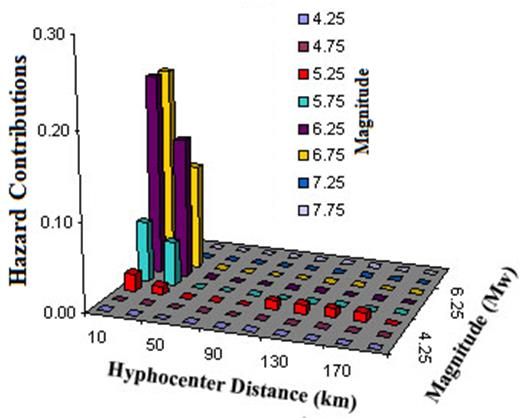Figure 9. Deaggregation result of Sleman.

6.3. Result of Amplification Process

Result of soil investigation for Sleman is in Figure 10. This Figure is correlation between Normal-Soil Penetration Tests (N-SPT) and soil layer depths. The data was the result of boring at the Sleman site.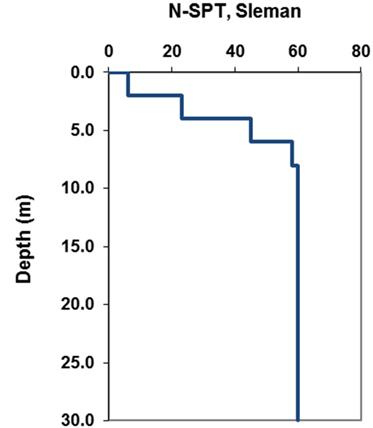Figure 10. Correlation of N-SPT and soil layer depth.

To obtain the soil type from Figure 10, the first is calculated average normal penetration test (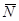) used a following formula (UBC1997):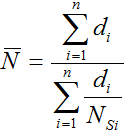(14)

where d is thickness of soil layer and NSi is N-SPT of each layer.

For Sleman site the average of N-SPT is= 34.6467. If this value plot to the Table 2a is obtained the soil type is SD. Based on PGA = 0.1976g and soil type SD, can be drawn the response spectra design of UBC 1997 for Sleman as in Figure 11. Response spectra Figure 11 is utilized as a target spectrum in spectral matching process.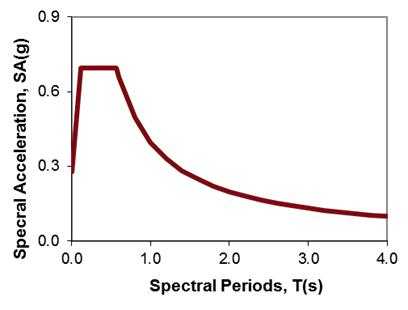Figure 11. Spectral design of Sleman (UBC 1997).

6.4. Result of Spectral Matching

From the deaggregation result is acquired magnitude and hypocenter distance that contribute most to the hazard in Sleman i.e. M = 6.3 and R = 19 km. Based on these magnitude and distance, the earthquake that approximate to the quantity is earthquake of Imperial Valley 1979, El Centro Array 01 Figure 12 with M = 6.53, rupture distance Rrup = 21.68 km, and soil site condition with Vs30 = 237.33 m/s (site type SD, UBC1997, Table 2b).

Actual spectrum of the ATH Figure 12 is in Figure 13. Spectral matching to obtain earthquake ATH for Sleman is carried out based on actual ATH (Figure 12) and target spectrum (Figure 11). Result of spectral matching is a scaling factor α = 2.6753. Also result of matching can be seen in Figure 14 and 15.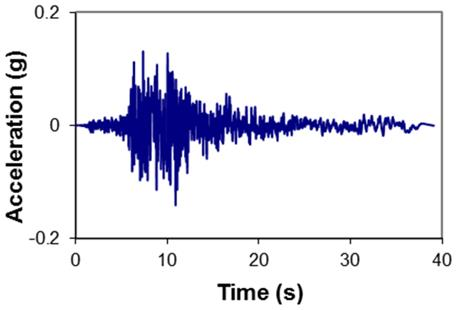Figure 12. ATH of Imperial Valley 1979, as actual ATH (accessed from http://ngawest2.berkeley.edu/,27 July 2015).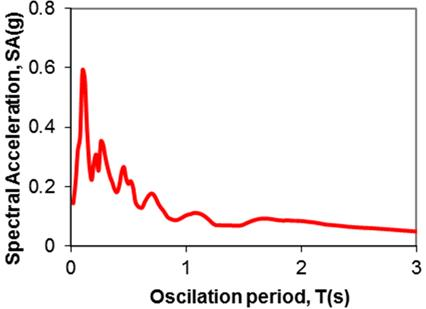Figure 13. Response spectra of ATH, Imperial Valley Earthquake 1979, El Centro array 01.

Matching spectrum in Figure 14 is acquired based on multiplying between response spectra Figure 13 and scaling factor a = 2.6753.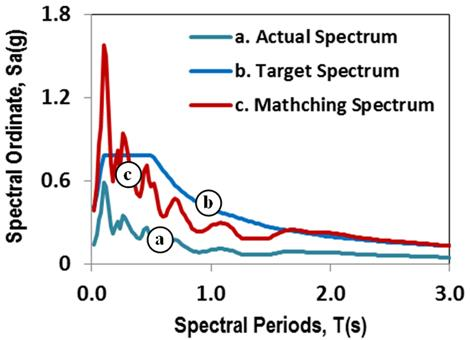Figure 14. Visual comparison of actual, target, and matching spectrum.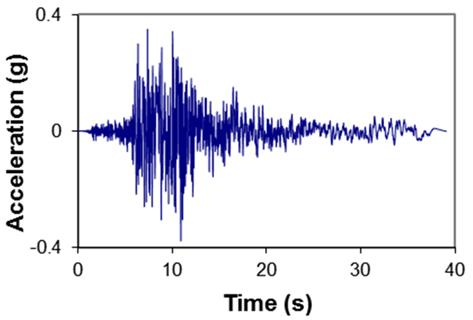Figure 15. Scaling earthquake ATH.

Scaling earthquake acceleration time history in Figure 15 is obtained from multiplying between ATH Figure 12 and scaling factor α = 2.6753.

The time history Figure 15 is the result of earthquake acceleration time history development for Sleman. Matching spectrum in Figure 14, response spectra design of UBC 1997 Figure 11 can be used as response spectra design for Sleman.

7. Discussion

The earthquake acceleration time history for Sleman district has been obtained in Figure 15. That time history can be utilized to design the tall building in the district with high more than 40 m. In addition, the result is response spectrum design (the target spectrum and matching spectrum) in Figure 14. Those response spectra can be used as basis to design the story building with high up to 40 m.

The PSHA, UBC 1997 code, and spectral matching in time domain are the methods to generate ground shaking in a site for the future, caused by earthquake. The results of these methods are the spectrum and ATH as shown in Figure 14 and 15. Figure 14 show that the matching spectrum adjusted to the target spectrum, because multiple between actual spectrum and scaling factor. Since value of matching spectrum ordinate increase is caused by scaling factor, there is no frequency contain change in the matching process. So there is no frequency contains change from the actual ATH Figure 12 to be the matching ATH Figure 15.

Because it has been figured out that the target spectrum is appropriate to the site, then the ATH with spectrum that was matched to the target spectrum has to be appropriate to the site.

8. Conclusion

The earthquake acceleration time history has been acquired for Sleman. The time history can be employed by the goverment of Sleman District as a basis to design tall building. Spectral matching in time domain gave an acceleration time history with frequency contain equal to the frequency contain of the actual time history. Because the target spectrum is assumed appropriate to the site, then the acceleration time history result also appropriate to the site.

References

1. Atkinson, G.M., Boore, D.M, Empirical Ground-Motion Relations for Subduction Zone Earthquakes and Their Application to Cascadia and Other Regions, Bull. Seismol. Soc. Am, Vol. 93, no. 4, 2003, pp. 1703-1729.
2. Boore D. M. dan Atkinson G.M. (2007), Ground Motion Relations for Geometric Mean Horizontal Component of Peak and Spectral Ground Motion Parameters, PEER Report 2007, Pacific Earthquake Engineering Research Center, College of Engineering University of California, Berkeley, May 2007, California, USA.
3. Gutenberg, B. and Richter, C., Frequency of Earhquakes in California, Bull. Seismol. Soc. Am., Vol. 34, 1944, pp. 185–188.
4. Kramer S.L. (1996), Geotechnical Earthquake Engineering. Prentice Hall:New Jersey.
5. Makrup L. 2009. Pengembangan Peta Deagregasi Hazard untuk Indonesia Melalui Pembuatan Software dengan Pemodelan Sumber Gempa Tiga Dimensi, Dissertation, Institute of Technology Bandung, Indonesia.
6. Makrup L., Irsyam M, Sengara I.W, and Hendriyawan (2015). Numerical Solution of the Total Probability Theorem in a Three Diensional Earthquake Source Domain for Developing Seismic Hazard Map and Hazard Spectrum, American Journal of Civil Engineering and Architecture, 2015, Vol. 3, No. 5, 158-164,DOI:10.12691/ajcea-3-5-2.
7. McGuire, (1996). R.K., Probabilistic Seismic Hazard Analysis and Design Earthquakes: Closing the Loop, Bull. Seismol. Soc. Am., Vol. 85, no. 5, 1995, pp. 1275-1284.
8. Nicolaou.A.S. (1998), A GIS Platform for Earthquake Risk Analysis. A dissertation submitted to the Faculty of the Graduate School of State University of New York at Buffalo USA in partial fulfillment of the requirement for the degree of Doctor of Philosophy, August.
9. Petersen M.D., Harmsen S., Mueller C., Haller K., Dewey J., Luco N., Crone A., Rukstales K., dan Lidke D., (2008), Probabilistic seismic hazard for Sooutheast Assia. International Conference of Earthquake Engineering and Disaster Mitigation, Jakarta, April 14, 15, 2008.
10. Sadigh K., Chang C.Y., Egan J.A., Makdisi F., dan Young R.R., (1997), Attenuation Relationships for shallow Crustal Earthquake Based on California Strong Motion Data, Seismological Research Letters, Volume 68 Januari/Pebruary 1997, Seismological Society of America.
11. UBC (1997), Uniform Building CodeTM, Volume 2, Structural Engineering Design Provisions, International Conference of Building Officials, 5360 WORKMAN MILL ROAD, WHITTIER, CALIFORNIA 90601-2298, PRINTED IN USA
12. Well D.L. and Coppersmith (1994), New Empirical Relation Among Magnitude, Rupture Length, Rupture Width, Rupture Area, and Surface Displacement, Bulletin of Seismological Society of America, Vol. 84, No.4, pp. 974-1002.
13. Youngs, R.R., Chiou, S. J, dan Silva W.J., (1997), Strong Ground Motion Attenuation Relationships for Subduction Zone Earthquakes, Seismological Research Letters, Volume 68 Januari/Pebruary 1997, Seismological Society of America.
14. Youngs, R. R. dan Coppersmith, K. J. (1985), Implications of Fault Slip Rates and Earthquake Recurrence Models to probabilistic Seismic Hazard Estimates, Bulletin of the Seismological Society of America, Vol. 75, No. 4, pp. 939-964.

 Contents 1. 2. 2.1. 2.2. 3. 4. 5. 6. 6.1. 6.2. 6.3. 6.4. 7. 8.
Article ToolsAbstractPDF(539K)